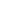this page is updated regularly
last update: 1 Apr 2018
contacts:mail us here2630 HP part numbers available
HP Components Part Numbers have two numerical sections splitted by dash. Each section has four digits.

Select here a requested HP Part Number and press "Go" button.

 HP Part Number: 1 = 25, MainCat = 0490 04902 = 25, MainCat = 1810 18103 = 25, MainCat = 1813 18134 = 25, MainCat = 1816 18165 = 25, MainCat = 1818 18186 = 25, MainCat = 1820 18207 = 25, MainCat = 1821 18218 = 25, MainCat = 1826 18269 = 25, MainCat = 1850 185010 = 25, MainCat = 1851 185111 = 25, MainCat = 1853 185312 = 25, MainCat = 1854 185413 = 25, MainCat = 1855 185514 = 25, MainCat = 1858 185815 = 25, MainCat = 1884 188416 = 25, MainCat = 1900 190017 = 25, MainCat = 1901 190118 = 25, MainCat = 1902 190219 = 25, MainCat = 1906 190620 = 25, MainCat = 1910 191021 = 25, MainCat = 1911 191122 = 25, MainCat = 1912 191223 = 25, MainCat = 1970 197024 = 25, MainCat = 2140 214025 = 25, MainCat = 5088 5088 - 0 1 2 3 4 5 6 7 8 9 0 1 2 3 4 5 6 7 8 9 0 1 2 3 4 5 6 7 8 9 0 1 2 3 4 5 6 7 8 9